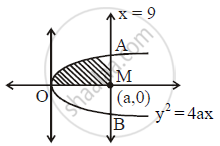# Find the area bounded by the curve y^2 = 4ax, x-axis and the lines x = 0 and x = a. - Mathematics and Statistics

Find the area bounded by the curve y2 = 4axx-axis and the lines x = 0 and x = a.

#### SolutionArea bounded = int_0^aydx

=int_0^asqrt(4ax)dx

=2sqrtaint_0^ax^(1/2)dx

=2sqrta[(x^(1/2+1))/(1/2+1)]_0^a

=2sqrtaxx2/3xxa^(3/2)

=4/3a^(1/2+3/2)

=4/3a^2

Concept: Area of the Region Bounded by a Curve and a Line
Is there an error in this question or solution?